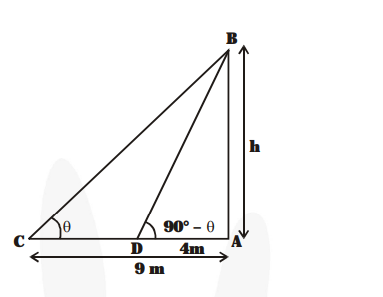# The angles of a elevation of the top of a tower from two points

Question:

The angles of a elevation of the top of a tower from two points at a distance of 4 m and 9 m from the base of the tower and in the same straight line with it are complementary. Prove that the height of the tower is 6 m.

Solution:

Let the tower be represented by AB in the figure.Let AB = h metres.

$\therefore$ In right $\Delta A B C$, we have

$\frac{\boldsymbol{A B}}{\boldsymbol{A C}}=\tan \theta$

$\Rightarrow \frac{\mathbf{h}}{\mathbf{9}}=\tan \theta$   ...(1)

In right $\triangle \mathrm{ABD}$, we have

$\frac{\mathbf{A B}}{\mathbf{A D}}=\tan \left(90^{\circ}-\theta\right)=\cot \theta$

$\Rightarrow \frac{\mathbf{h}}{\mathbf{4}}=\cot \theta$   ...(2)

Multiplying (1) and (2), we get

$\frac{\mathbf{h}}{\mathbf{9}} \times \frac{\mathbf{h}}{\mathbf{4}}=\tan \theta \times \cot \theta=1 \quad[\because \tan \theta \times \cot \theta=1]$

$\Rightarrow \frac{\mathbf{h}^{2}}{\mathbf{3 6}}=1 \Rightarrow \mathrm{h}^{2}=36$

$\Rightarrow \mathrm{h}=\pm 6 \mathrm{~m} \quad \therefore \quad \mathrm{h}=6 \mathrm{~m}$

$[\because$ Height is positive only $]$

Thus, the height of the tower is $6 \mathrm{~m}$.